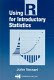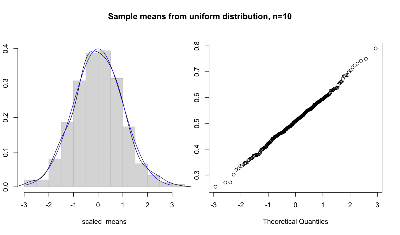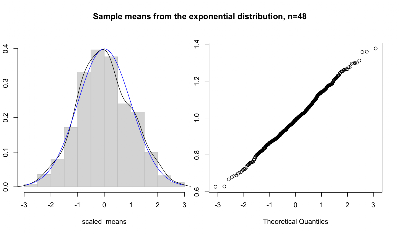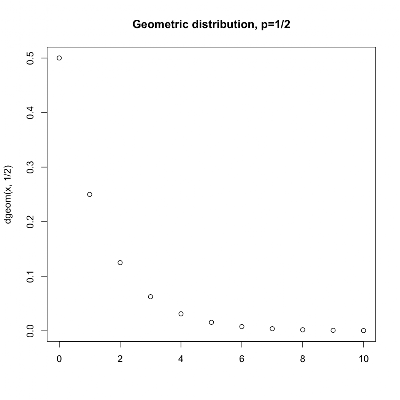## Monday, March 21, 2011

### Using R for Introductory Statistics 6, SimulationsR can easily generate random samples from a whole library of probability distributions. We might want to do this to gain insight into the distribution's shape and properties. A tricky aspect of statistics is that results like the central limit theorem come with caveats, such as "...for sufficiently large n...". Getting a feel for how large is sufficiently large, or better yet, testing it, is the purpose of the simulations in chapter 6 of John Verzani's Using R for Introductory Statistics.

The central limit theorem says that the sample mean drawn from any parent population will tend towards being normally distributed as n, the size of the sample, grows larger. The sample size needed so that the normal distribution reasonably approximates the sample mean varies from one type of distribution to another.

A general form of a test that allows us to eyeball the accuracy of the approximation would look something like the code below, which generates and takes the mean of 300 samples drawn from any of R's random number generation functions (rnorm, runif, rbinom, rexp, rt, rgeom, etc.), scaling the sample means to a mean of zero and standard deviation of one. In a two panel plot the function first plots a histogram and a density curve along with the standard normal curve for comparison, and then does a quantile-quantile plot comparing the sample to a normal distribution.

```plot_sample_means <- function(f_sample, n, m=300,title="Histogram", ...) {

# define a vector to hold our sample means
means <- double(0)

# generate 300 samples of size n and store their means
for(i in 1:m) means[i] = mean(f_sample(n,...))

# scale sample means to plot against standard normal
scaled_means <- scale(means)

# set up a two panel plot
par(mfrow=c(1,2))
par(mar=c(5,2,5,1)+0.1)

# plot histogram and density of scaled means
hist(scaled_means, prob=T, col="light grey", border="grey", main=NULL, ylim=c(0,0.4))
lines(density(scaled_means))

# overlay the standard normal curve in blue for comparison

# adjust margins and draw the quantile-quantile plot
par(mar=c(5,1,5,2)+0.1)
qqnorm(means, main="")

# return margins to normal and go back to one panel
par(mar=c(5,4,4,2)+0.1)
par(mfrow=c(1,1))

par(omi=c(0,0,0.75,0))
title(paste(title, ", n=", n, sep=""), outer=T)
par(omi=c(0,0,0,0))

# return unscaled means (without printing)
return(invisible(means))
}
```

This function shows off some of the goodness that the R programming language adopts from the functional school of programming languages. First, note that it's a function that takes another function as an argument. Like most modern languages, R treats functions as first-class objects. Also note the use of the ellipsis to pass arbitrary arguments onward to the f_sample function. All of the r_ functions take n as a parameter. But, aside from that, the parameters differ. The ability to handle situations like this makes R's ellipsis a bit more powerful than the similar looking varargs functions in C-style languages.

Note what happens for n=1. In this case, the sample mean is just a sample. So, as n increases, we can morph any distribution into the normal. For instance, here's a series of plots of sample means drawn from the uniform distribution. We start at n=1, which looks flat as expected. At n=2 we already get a pretty good fit to the normal curve, except at the tails. The n=10 case closely fits to the normal.

```> plot_sample_means(runif, n=1, title="Sample means from uniform distribution")
``````> plot_sample_means(runif, n=2, title="Sample means from uniform distribution")
``````> plot_sample_means(runif, n=10, title="Sample means from uniform distribution")
```Trying the same trick with other distributions yields different results. The exponential distribution takes a while to loose it's skew. Here are plots for n=6, 12, and 48.

```> plot_sample_means(rexp, n=6, title="Sample means from the exponential distribution", rate=1)
``````> plot_sample_means(rexp, n=12, title="Sample means from the exponential distribution", rate=1)
``````> plot_sample_means(rexp, n=48, title="Sample means from the exponential distribution", rate=1)
```I'm guessing that Verzani's purpose with the simulations in this chapter is for the reader to get some intuitive sense of the properties of these distributions and their relationships, as an alternative to delving too deeply into theory. This lets us stick to applying statistical tools, while giving us some handle on how things might go wrong.

## Friday, March 18, 2011

### Indexes are good

I have a MySQL database, on top of which we're trying to do a little data mining. I'm going to transpose domains, to protect the guilty. So, here's the (transposed) schema:

```mysql> describe shoppingbaskets;
+-------------------+---------+
| Field             | Type    |
+-------------------+---------+
| id                | int(11) |
...etc...
| count_items       | int(11) |
+-------------------+---------+

+-------------------+---------+
| Field             | Type    |
+-------------------+---------+
| item_id           | int(11) |
+-------------------+---------+

mysql> describe items;
+-------------------+---------+
| Field             | Type    |
+-------------------+---------+
| id                | int(11) |
...etc...
+-------------------+---------+
```

It was kinda pokey to count the number of items in a basket on the fly, so I added the count_items column to shoppingbaskets to cache that information. To populate that column, I cooked up a little query like so:

`mysql> update shoppingbaskets b set count_items = (select count(*) from shoppingbasket_items bi where bi.shoppingbasket_id=b.id);`

So, I popped that in and waited... Looking at the query, it seems to me that it would be linear in the number of shopping baskets. But, I guess it's scanning all of the items for each basket.

Hmmm, how long was this going to take? I tried a few small test cases and came up with this bit of R code:

```> qt = read.table('query_timing.txt', sep="\t", quote="", header=T)
> qt
n seconds
1   1    0.57
2   2    6.71
3  10   55.20
4  11   61.76
5  20  116.72
6  50  297.83
7  80  481.17
8 100  606.04
9 118  709.70
> plot(seconds ~ n, data=qt, main="Time to count items in n baskets")
> model <- lm(seconds ~ n, data=qt)
> model

Call:
lm(formula = seconds ~ n, data = qt)

Coefficients:
(Intercept)            n
-5.331        6.081

> abline(model, col='blue', lty=2)
```Nicely linear, OK. But at 6 seconds per basket and 155,588 baskets, we're looking at 10 days. mysqladmin shutdown! Ok, now let's start up a brain cell or two.

#### Duh... index

```create index idx_by_shoppingbasket_id on shoppingbasket_items (shoppingbasket_id);
```

Now filling the entire count_items column for all 155,588 rows takes 11.06 seconds. But, now that we can quickly count items on the fly, why bother caching the counts? Column dropped. Problem solved.

Conclusion: Indexes are good. Note to self: Don't forget to think now and then, you knucklehead. This web page describes exactly my situation.

## Sunday, March 13, 2011

### Using R for Introductory Statistics, The Geometric distribution

We've already seen two discrete probability distributions, the binomial and the hypergeometric. The binomial distribution describes the number of successes in a series of independent trials with replacement. The hypergeometric distribution describes the number of successes in a series of independent trials without replacement. Chapter 6 of Using R introduces the geometric distribution - the time to first success in a series of independent trials.Specifically, the probability the first success occurs after k failures is:Note that this formulation is consistent with R's [r|d|p|q]geom functions, while the book defines the distribution slightly differently as the probability that the first success occurs on the kth trial, changing the formula to:We'll use the first formula, so k ∈ 0,1,2,..., where 0 means no failures - success on the first try. The intuition is that the probability of failure is (1-p), so the probability of k failure is (1-p) to the kth power.

Let's generate 100 random samplings where the probability of success on any given trial is 1/2, like we were repeatedly flipping a coin and recording how many heads we got before we got a tail.

```> sample <- rgeom(100, 1/2)
> summary(sample)
Min. 1st Qu.  Median    Mean 3rd Qu.    Max.
0.0     0.0     0.0     0.9     1.0     5.0
> sd(sample)
 1.184922
> hist(sample, breaks=seq(-0.5,6.5, 1), col='light grey', border='grey', xlab="")
```As expected, we get success on the first try about half the time, and the frequency drops in half for every increment of k after that.The median is 0, because about 1/2 the samples are 0. The mean is, of course, higher because of the one-sidedness of the distribution. The mean of our sample is 0.9, which is not too far from the expected value of 1. Likewise, the standard deviation is not far from the theoretical value of √2 or 1.414214.

This is part of an ultra-slow-motion reading of John Verzani's Using R for Introductory Statistics. Notes on previous chapters can be found here:

Chapters 1 and 2

Chapter 3

Chapter 4

Chapter 5

## Tuesday, March 01, 2011

### Learning data science skillsAccording to Hal Varian and just about everyone these days, the hot skills to have are some combination of programming, statistics, machine learning, and visualization. Here are a pile of resources that'll help you get some mad data science skills.

#### Programming

There seems to be a few main platforms widely used for data intensive programming. R, is a statistical environment that is to statisticians what MatLab is to engineers. It's a weird beast, but it's open source and very powerful, plus has a great community. Python also makes a strong showing, with the help of NumPy, SciPy and matplotlib. An intriguing new entry is the combination of the Lisp dialect Clojure and Incanter. All these tools mix numerical libraries with functional and scripting programming styles in varying proportions. You'll also want to look into Hadoop, to do your big data analytics map-reduce style in the cloud.

#### Statistics

• John Verzani's Using R for Introductory Statistics, which I'm working my way through.
• #### Machine Learning

• Toby Segaran's Programming Collective Intelligence
• The Elements of Statistical Learning: Data Mining, Inference, and Prediction
• Bishop's Pattern Recognition and Machine Learning
• Machine Learning, Tom Mitchell
•#### Visualization

• Tufte's books, especially The Visual Display of Quantitative Information
• Processing, along with Ben Fry's book, Visualizing Data.
• Jeffrey Heer's papers, especially Software Design Patterns for Information Visualization. Heer is one of the creators of several toolkits: Prefuse, Flare and Protovis.
• 7 Classic Foundational Vis Papers and Seminal information visualization papers
• #### Classes

Starting on March 5 at the Hacker Dojo in Mountain View (CA), Mike Bowles and Patricia Hoffmann will present a course on Machine Learning where R will be the "lingua franca" for looking at homework problems, discussing them and comparing different solution approaches. The class will begin at the level of elementary probability and statistics and from that background survey a broad array of machine learning techniques including: Unsupervised Learning, Clustering Techniques, and Fault Detection.

R courses from Statistics.com

Feb 11:  Modeling in R (Sudha Purohit -- more details after the jump)
Mar 4:  Introduction to R - Data Handling (Paul Murrell)
Apr 15:  Programming in R (Hadley Wickham)
Apr 29:  Graphics in R (Paul Murrell)
May 20:  Introduction to R – Statistical Analysis (John Verzani)

Data bootcamp (slides and code) from the Strata Conference. Tutorials covering a handful of example problems using R and python.

• Plotting data on maps
• Classifying emails
• A classification problem in image analysis